Hi Mike, Can you please explain Question 11, Test 6, section 3 ? I know the parabola opens downward, but I’m confused after that. Thanks.

You’ve already got the first part—when you add a negative to the front of a parabola you’ll flip it vertically. Since this parabola begins facing up, the negative flips it so it faces down.

From there, I think it’s helpful to think about this in terms of how functions shift. For some function f(x) that’s graphed on the xy-plane:

• f(x)+1 ⇒ (graph moves UP one)
• f(x)–1 ⇒ (graph moves DOWN one)
• f(x+1) ⇒ (graph moves LEFT one)
• f(x–1) ⇒ (graph moves RIGHT one)

That applies here. You’re basically taking the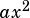function and applying a few shifts (and a flip). Check it out–if you start by assuming a function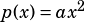, you can build the function in this question by shifting that original function: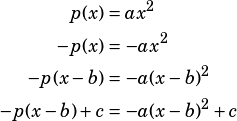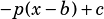flips the functionupside-down, then shifts it b units to the right and c units up.

If you hate everything I’ve just said, I have good news! You can also just memorize the vertex form of a parabola (which we basically just derived).

If you have a parabola in the form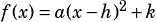, then you know it has its vertex atand that the sign of a tells you whether the parabola opens up or down. This question basically gives you the vertex form, only it uses b and c instead of h and k. Recognize that and you know right away that the parabola’s vertex is at.